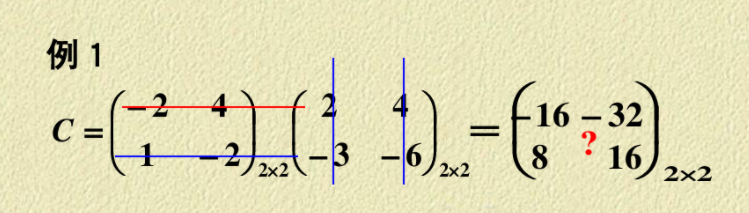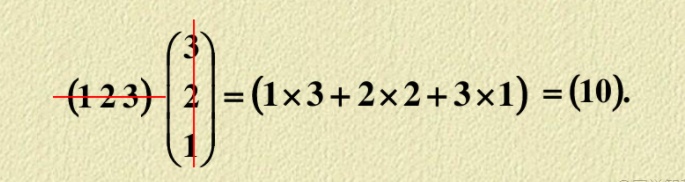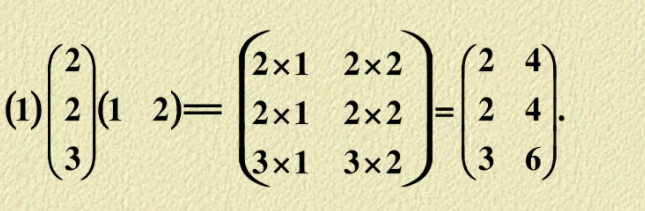# pta L1-048 矩阵A乘以B (15 分)

• 时间:
• 浏览:

就是a矩阵的每    乘以 b矩阵的每 两两相乘加起来L1-048 矩阵A乘以B (15 分)

### 输入样例1：

``````2 3
1 2 3
4 5 6
3 4
7 8 9 0
-1 -2 -3 -4
5 6 7 8``````

### 输出样例1：

``````2 4
20 22 24 16
53 58 63 28``````

### 输入样例2：

``````3 2
38 26
43 -5
0 17
3 2
-11 57
99 68
81 72``````

### 输出样例2：

``Error: 2 != 3``
``````//L1-048 矩阵A乘以B (15 分)
#include<iostream>
#include<stdio.h>
#include <string>
using namespace std;
int main()
{
int r,c,r1,c1;//行列
cin>>r>>c;
int a[r][c];
for(int i=0;i<r;i++){
for(int j=0;j<c;j++){
cin>>a[i][j];
}
}
//	for(int i=0;i<r;i++){
//		for(int j=0;j<c;j++){
//			cout<<a[i][j]<<" ";
//		}
//			cout<<endl;
//	}
cin>>r1>>c1;
int b[r1][c1];
for(int i=0;i<r1;i++){
for(int j=0;j<c1;j++){
cin>>b[i][j];
}
}
if(c!=r1){
string res = "Error: "+to_string(c)+" != "+to_string(r1);
cout<<res<<endl;
}else{
cout<<r<<" "<<c1<<endl;

//C是列，R是行。
//先是我的行然后你的列
//我的列跟你的行是一样的
for(int i=0;i<r;i++)
{
for(int j=0;j<c1;j++)
{
int sum=0;
for(int k=0;k<c;k++)
sum+=a[i][k]*b[k][j];
if(j<c1-1)
cout<<sum<<" ";
else
cout<<sum<<endl;
}
}
}
}
``````

2